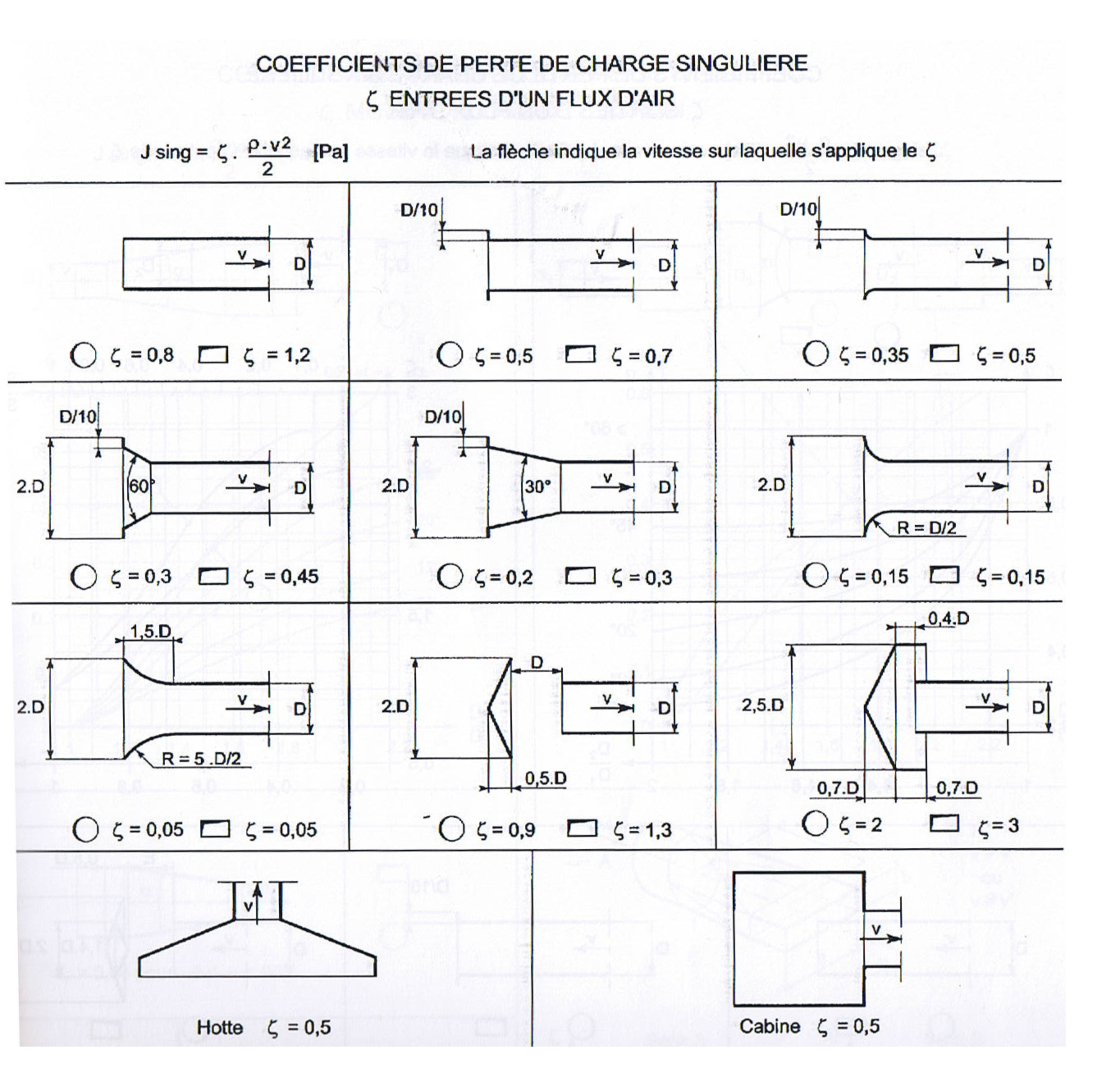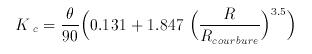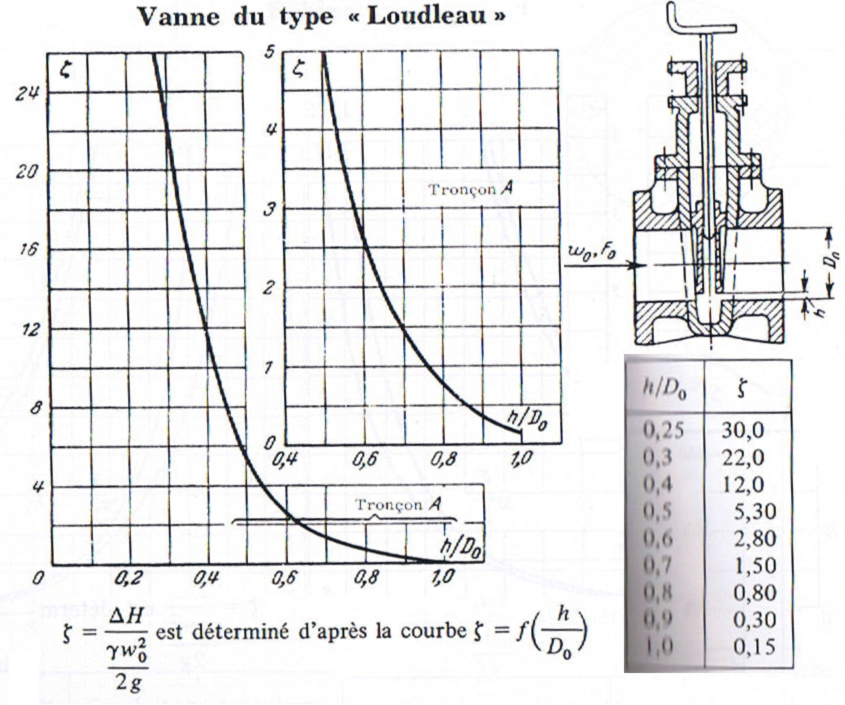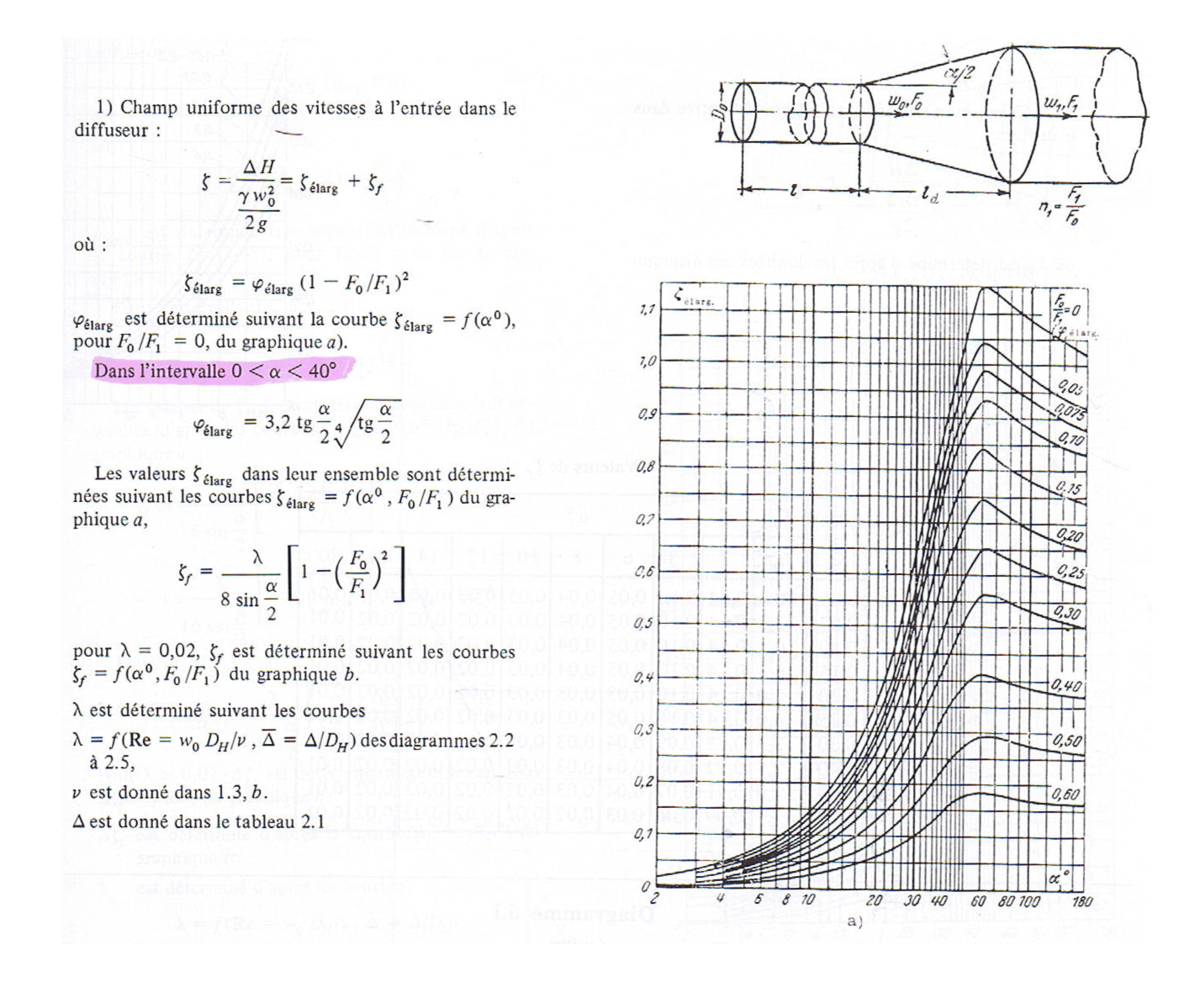automatic creation of common elements such as shrinkage, elbows, cones, valves, fittings

The Minor head losses elements editor of mecaflux pro3D, has an equation solver and a recording system by reading on curves. It is possible to use all forms of data sources of Minor head losses coefficients and store them in the library:

Minor head losses Coefficient Constant :
Head pressure loss in pipes fittings and networks elements :

There are two types of elements in a network:

1. Ducts or pipes that are regular elements whose loss is calculated according to the rate , roughness and Reynolds number with the Major head loss coefficient given by the equation Colebrook White
2. And all other elements that are singular. Depending on the characteristics of the singular element studied, Minor head loss is due to any pressure drop caused by an elbow, tee, valve, etc. and is usually expressed as some coefficient (K) of the velocity head. To learn more : methods for measuring Minor head loss coefficients.

Minor head loss coefficients greatly facilitate studies losses because it is applicable to all fluids(newtonians) and all flows (It is generally acknowledged for ventilation or hydraulic networks that we consider as Newtonian the fluids and that their compressibility can be neglected)

For modeling singular elements(like pipe fittings) in a network, Mecaflux Pro3D provides a system for automatic creation of common elements such as shrinkage, elbows, cones, valves, fittings... See the tutorial: Automatically insert usual Elements singular(like pipe fittings) in the network:

for more specific singular elements, Mecaflux Pro 3D provides fitting and singular element editor. This editor allow to input Minor head loss coefficients , and store this element in the library. Sources giving information on the Minor head loss coefficients are numerous.:

• Tables for an element in a precise configuration. :
•• Semi-empirical formulas linking the various characteristics of the element:
• Wiesbach formula for elbows : It is an expression of the Minor head loss coefficient as a function of the radius of curvature(R curvature), angle (thêta) and radius(R):• Tables from adjustment of elements like Valves:• Graphs of changes in the coefficient depending on the characteristics of shape:The diversity of expressions of coefficient loss is due to the multitude of cases encountered in the design of network system fluid. The element editor of mecaflux Pro 3D is suitable for the variety of expressions of the Minor head loss coefficient:

• Minor head loss coefficient constant:

Example of creating a singular element with a constant coefficient:

• Minor head loss coefficient as a function of a parameter:

Example of creating a singular element with a coefficient given in a graph or a mathematical expression: